Скачать презентацию PHYS 1443 Section 001 Lecture 17 Thursday

71dd680750fe9e8d45b9fd8744af99f1.ppt

• Количество слайдов: 21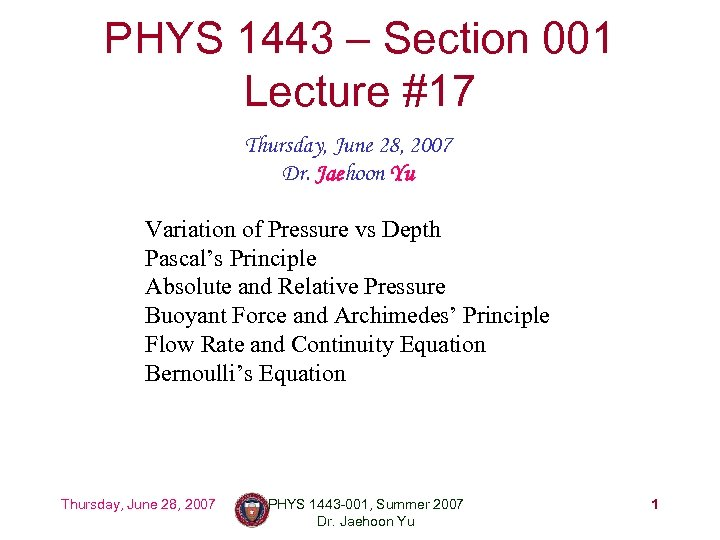PHYS 1443 – Section 001 Lecture #17 Thursday, June 28, 2007 Dr. Jaehoon Yu Variation of Pressure vs Depth Pascal’s Principle Absolute and Relative Pressure Buoyant Force and Archimedes’ Principle Flow Rate and Continuity Equation Bernoulli’s Equation Thursday, June 28, 2007 PHYS 1443 -001, Summer 2007 Dr. Jaehoon Yu 1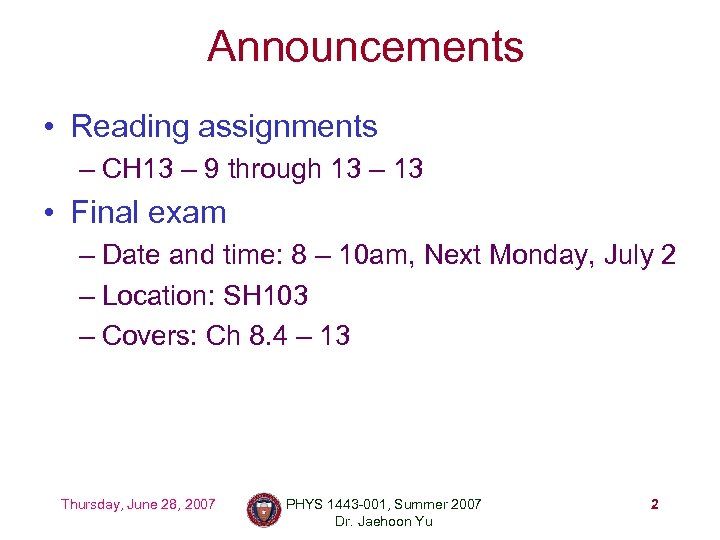Announcements • Reading assignments – CH 13 – 9 through 13 – 13 • Final exam – Date and time: 8 – 10 am, Next Monday, July 2 – Location: SH 103 – Covers: Ch 8. 4 – 13 Thursday, June 28, 2007 PHYS 1443 -001, Summer 2007 Dr. Jaehoon Yu 2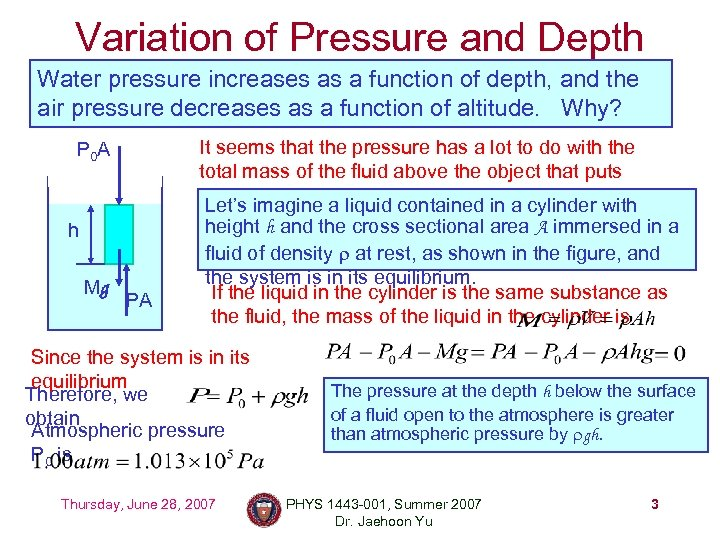Variation of Pressure and Depth Water pressure increases as a function of depth, and the air pressure decreases as a function of altitude. Why? It seems that the pressure has a lot to do with the total mass of the fluid above the object that puts weight on the object. contained in a cylinder with Let’s imagine a liquid P 0 A h Mg PA height h and the cross sectional area A immersed in a fluid of density r at rest, as shown in the figure, and the system is in its equilibrium. If the liquid in the cylinder is the same substance as the fluid, the mass of the liquid in the cylinder is Since the system is in its equilibrium Therefore, we obtain Atmospheric pressure P 0 is Thursday, June 28, 2007 The pressure at the depth h below the surface of a fluid open to the atmosphere is greater than atmospheric pressure by rgh. PHYS 1443 -001, Summer 2007 Dr. Jaehoon Yu 3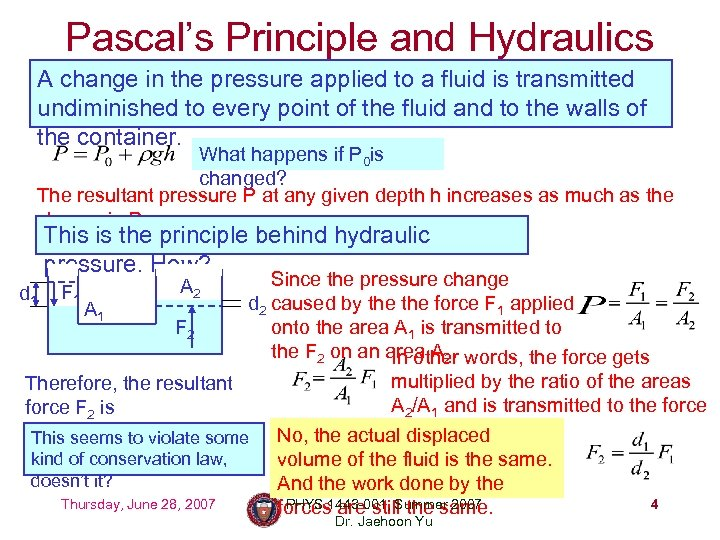Pascal’s Principle and Hydraulics A change in the pressure applied to a fluid is transmitted undiminished to every point of the fluid and to the walls of the container. What happens if P 0 is changed? The resultant pressure P at any given depth h increases as much as the change in P 0. This is the principle behind hydraulic pressure. How? Since the pressure change d 2 caused by the force F 1 applied A 1 F 2 onto the area A 1 is transmitted to the F 2 on an area A 2. words, the force gets In other multiplied by the ratio of the areas Therefore, the resultant A 2/A 1 and is transmitted to the force F 2 is F displaced No, the actual 2 on the surface. This seems to violate some kind of conservation law, volume of the fluid is the same. doesn’t it? And the work done by the Thursday, June 28, 2007 PHYS 1443 -001, Summer 2007 4 forces are still the same. d 1 F 1 A 2 Dr. Jaehoon Yu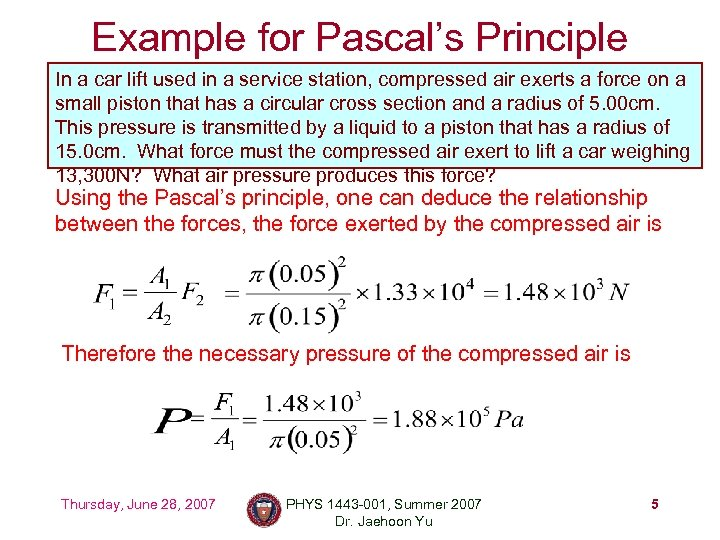Example for Pascal’s Principle In a car lift used in a service station, compressed air exerts a force on a small piston that has a circular cross section and a radius of 5. 00 cm. This pressure is transmitted by a liquid to a piston that has a radius of 15. 0 cm. What force must the compressed air exert to lift a car weighing 13, 300 N? What air pressure produces this force? Using the Pascal’s principle, one can deduce the relationship between the forces, the force exerted by the compressed air is Therefore the necessary pressure of the compressed air is Thursday, June 28, 2007 PHYS 1443 -001, Summer 2007 Dr. Jaehoon Yu 5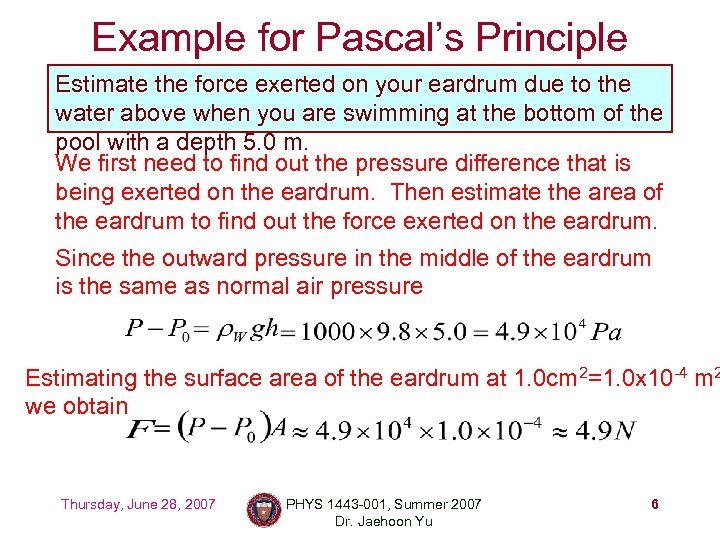Example for Pascal’s Principle Estimate the force exerted on your eardrum due to the water above when you are swimming at the bottom of the pool with a depth 5. 0 m. We first need to find out the pressure difference that is being exerted on the eardrum. Then estimate the area of the eardrum to find out the force exerted on the eardrum. Since the outward pressure in the middle of the eardrum is the same as normal air pressure Estimating the surface area of the eardrum at 1. 0 cm 2=1. 0 x 10 -4 m 2 we obtain Thursday, June 28, 2007 PHYS 1443 -001, Summer 2007 Dr. Jaehoon Yu 6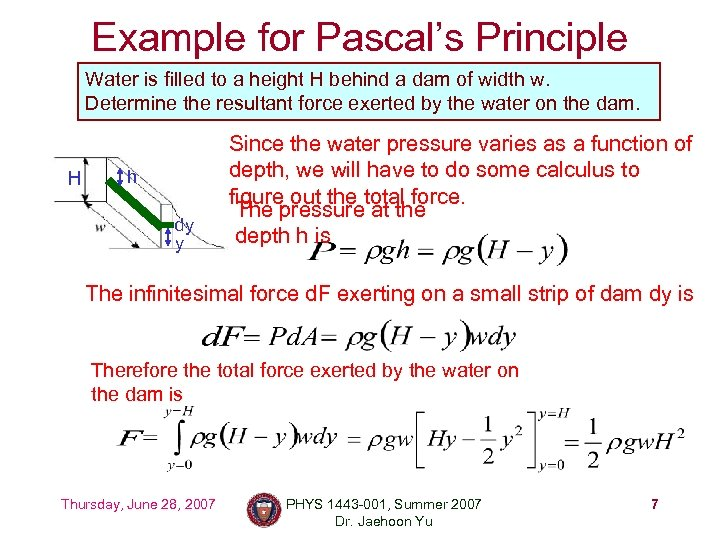Example for Pascal’s Principle Water is filled to a height H behind a dam of width w. Determine the resultant force exerted by the water on the dam. H h dy y Since the water pressure varies as a function of depth, we will have to do some calculus to figure out the total force. The pressure at the depth h is The infinitesimal force d. F exerting on a small strip of dam dy is Therefore the total force exerted by the water on the dam is Thursday, June 28, 2007 PHYS 1443 -001, Summer 2007 Dr. Jaehoon Yu 7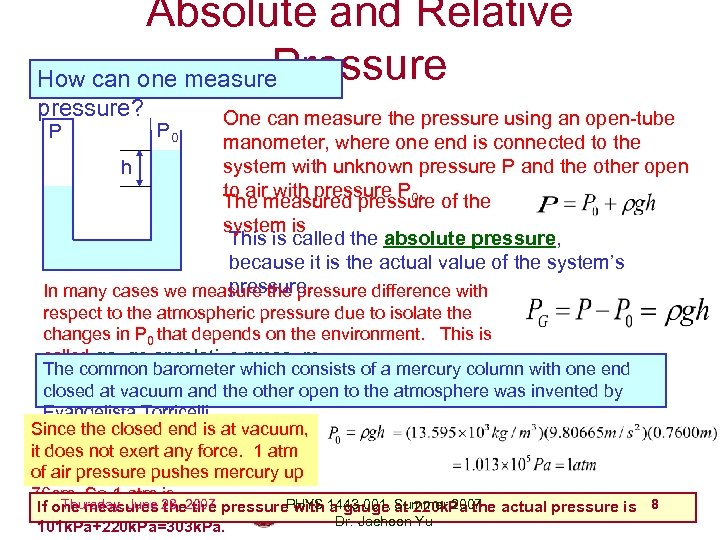Absolute and Relative Pressure How can one measure pressure? One can measure the pressure using an open-tube manometer, where one end is connected to the system with unknown pressure P and the other open h to air with pressure P 0. The measured pressure of the system is This is called the absolute pressure, because it is the actual value of the system’s pressure. In many cases we measure the pressure difference with P P 0 respect to the atmospheric pressure due to isolate the changes in P 0 that depends on the environment. This is called gauge or relative pressure. The common barometer which consists of a mercury column with one end closed at vacuum and the other open to the atmosphere was invented by Evangelista Torricelli. Since the closed end is at vacuum, it does not exert any force. 1 atm of air pressure pushes mercury up 76 cm. So 1 atm is Thursday, June the tire If one measures 28, 2007 pressure. PHYS 1443 -001, at 220 k. Pa the actual pressure is 8 with a gauge Summer 2007 Dr. Jaehoon Yu 101 k. Pa+220 k. Pa=303 k. Pa.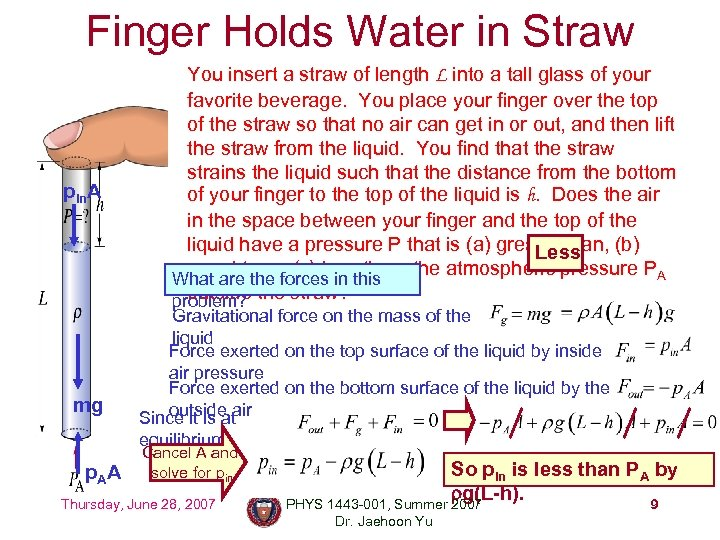Finger Holds Water in Straw pin. A mg You insert a straw of length L into a tall glass of your favorite beverage. You place your finger over the top of the straw so that no air can get in or out, and then lift the straw from the liquid. You find that the straw strains the liquid such that the distance from the bottom of your finger to the top of the liquid is h. Does the air in the space between your finger and the top of the liquid have a pressure P that is (a) greater than, (b) Less equal to, or (c) less than, the atmospheric pressure PA What are the forces in this outside problem? the straw? Gravitational force on the mass of the liquid Force exerted on the top surface of the liquid by inside air pressure Force exerted on the bottom surface of the liquid by the outside air Since it is at equilibrium p. AA Cancel A and solve for pin Thursday, June 28, 2007 So pin is less than PA by rg(L-h). PHYS 1443 -001, Summer 2007 9 Dr. Jaehoon Yu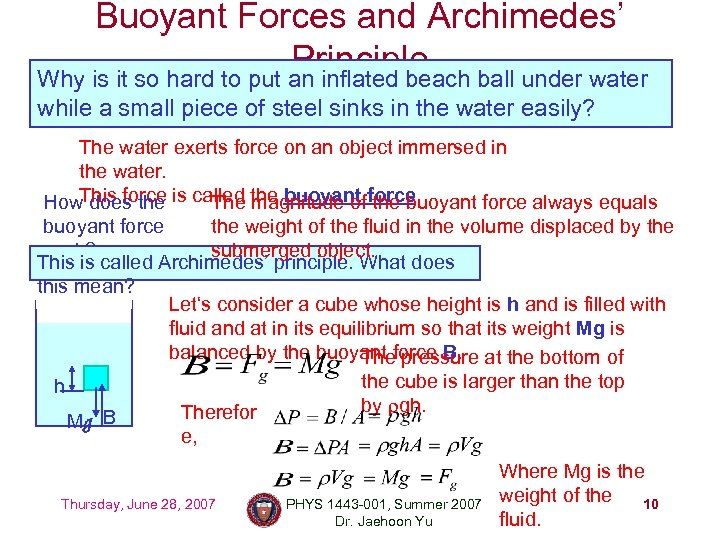Buoyant Forces and Archimedes’ Principle ball under water Why is it so hard to put an inflated beach while a small piece of steel sinks in the water easily? The water exerts force on an object immersed in the water. This force How does the is called the buoyant force. The magnitude of the buoyant force always equals buoyant force the weight of the fluid in the volume displaced by the work? submerged object. This is called Archimedes’ principle. What does this mean? Let‘s consider a cube whose height is h and is filled with fluid and at in its equilibrium so that its weight Mg is balanced by the buoyant force B. at the bottom of The pressure the cube is larger than the top h by rgh. Therefor B Mg e, Thursday, June 28, 2007 PHYS 1443 -001, Summer 2007 Dr. Jaehoon Yu Where Mg is the weight of the 10 fluid.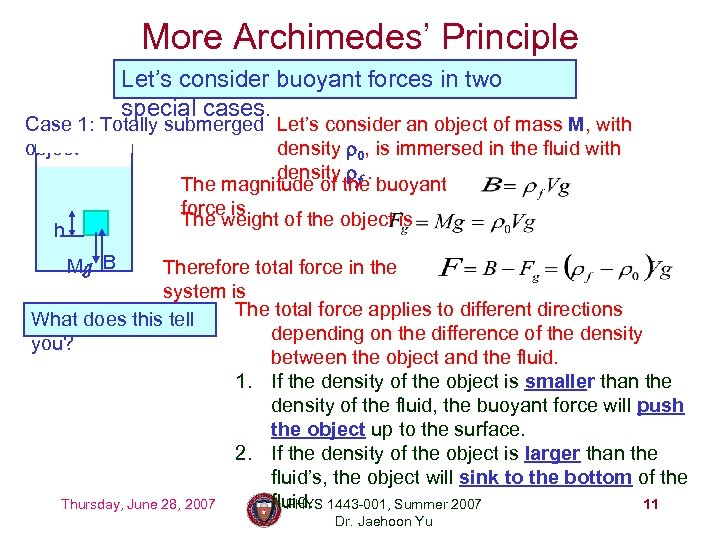More Archimedes’ Principle Let’s consider buoyant forces in two special cases. Case 1: Totally submerged Let’s consider an object of mass M, with object density r 0, is immersed in the fluid with density rf. The magnitude of the buoyant force is The weight of the object is h Mg B Therefore total force in the system is The total force applies to different directions What does this tell depending on the difference of the density you? between the object and the fluid. 1. If the density of the object is smaller than the density of the fluid, the buoyant force will push the object up to the surface. 2. If the density of the object is larger than the fluid’s, the object will sink to the bottom of the fluid. 1443 -001, Summer 2007 Thursday, June 28, 2007 PHYS 11 Dr. Jaehoon Yu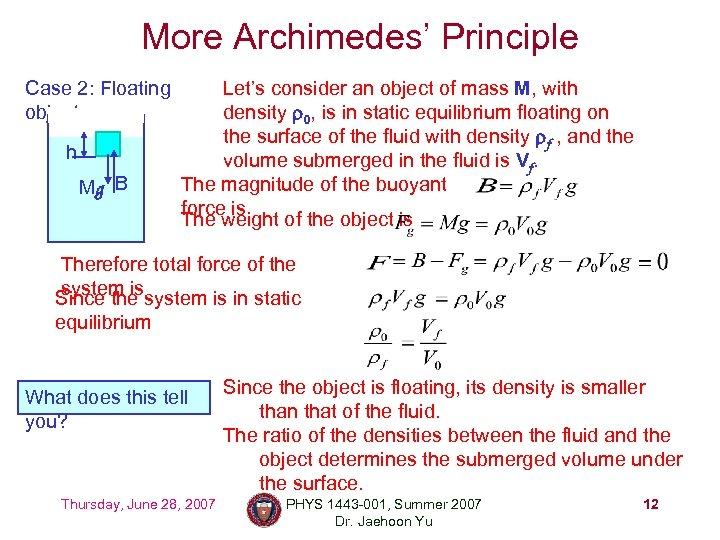More Archimedes’ Principle Case 2: Floating object h Mg B Let’s consider an object of mass M, with density r 0, is in static equilibrium floating on the surface of the fluid with density rf , and the volume submerged in the fluid is Vf. The magnitude of the buoyant force is The weight of the object is Therefore total force of the system is Since the system is in static equilibrium What does this tell you? Thursday, June 28, 2007 Since the object is floating, its density is smaller than that of the fluid. The ratio of the densities between the fluid and the object determines the submerged volume under the surface. PHYS 1443 -001, Summer 2007 Dr. Jaehoon Yu 12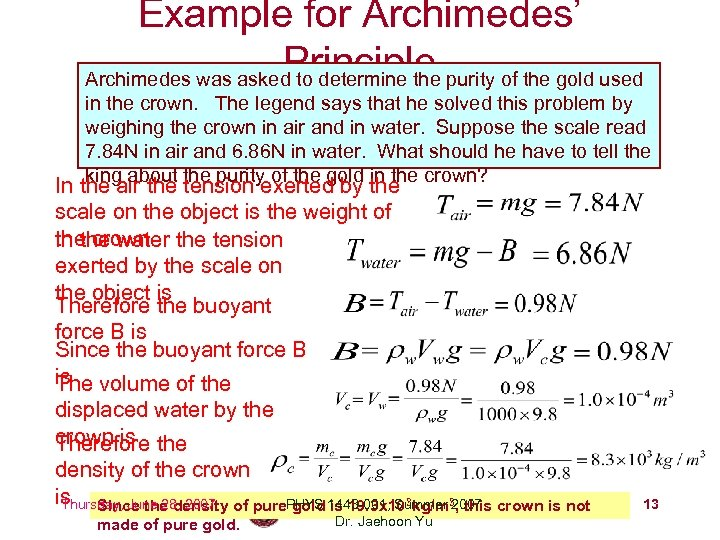Example for Archimedes’ Principle Archimedes was asked to determine the purity of the gold used in the crown. The legend says that he solved this problem by weighing the crown in air and in water. Suppose the scale read 7. 84 N in air and 6. 86 N in water. What should he have to tell the king about the purity of the gold in the crown? In the air the tension exerted by the scale on the object is the weight of the crown the tension In the water exerted by the scale on the object is Therefore the buoyant force B is Since the buoyant force B is volume of the The displaced water by the crown is the Therefore density of the crown is Since the density of pure. PHYS 1443 -001, Summer 32007 crown is not Thursday, June 28, 2007 gold is 19. 3 x 103 kg/m , this made of pure gold. Dr. Jaehoon Yu 13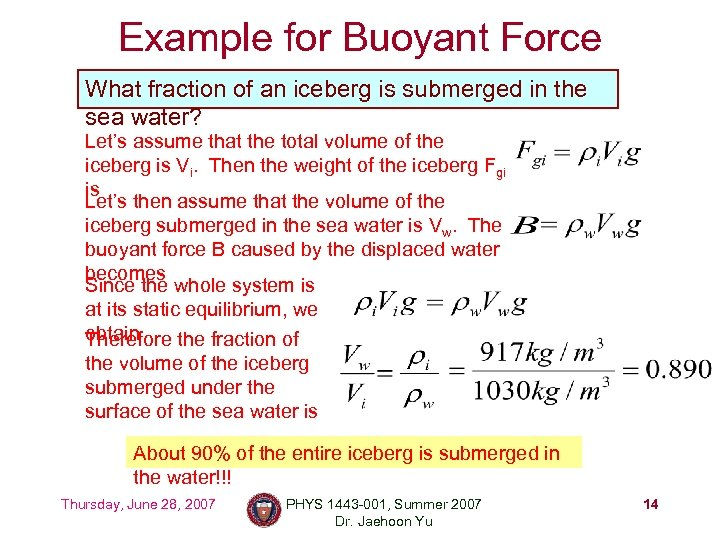Example for Buoyant Force What fraction of an iceberg is submerged in the sea water? Let’s assume that the total volume of the iceberg is Vi. Then the weight of the iceberg Fgi is Let’s then assume that the volume of the iceberg submerged in the sea water is Vw. The buoyant force B caused by the displaced water becomes Since the whole system is at its static equilibrium, we obtain Therefore the fraction of the volume of the iceberg submerged under the surface of the sea water is About 90% of the entire iceberg is submerged in the water!!! Thursday, June 28, 2007 PHYS 1443 -001, Summer 2007 Dr. Jaehoon Yu 14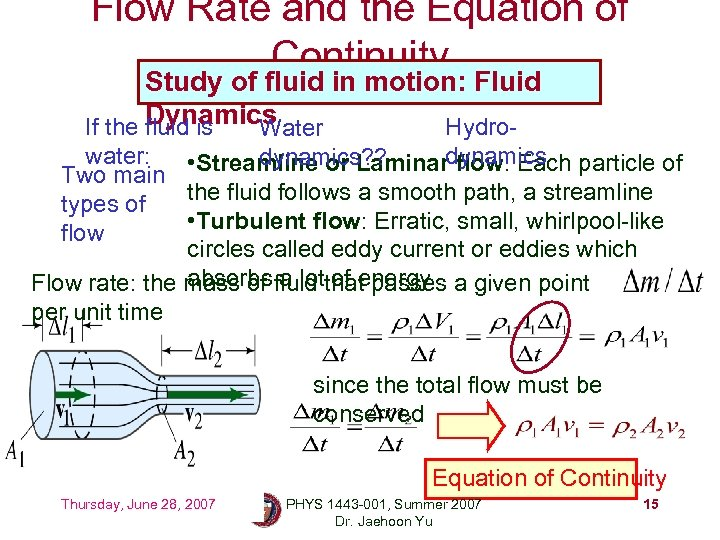Flow Rate and the Equation of Continuity Study of fluid in motion: Fluid Dynamics If the fluid is Hydro. Water water: dynamics? ? • Streamline or Laminardynamics particle of flow: Each Two main the fluid follows a smooth path, a streamline types of • Turbulent flow: Erratic, small, whirlpool-like flow circles called eddy current or eddies which absorbs a lot of energy Flow rate: the mass of fluid that passes a given point per unit time since the total flow must be conserved Equation of Continuity Thursday, June 28, 2007 PHYS 1443 -001, Summer 2007 Dr. Jaehoon Yu 15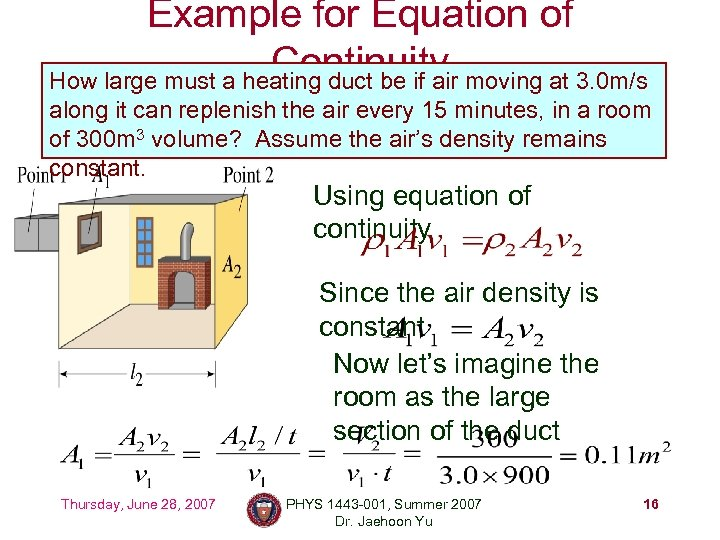Example for Equation of Continuity moving at 3. 0 m/s How large must a heating duct be if air along it can replenish the air every 15 minutes, in a room of 300 m 3 volume? Assume the air’s density remains constant. Using equation of continuity Since the air density is constant Now let’s imagine the room as the large section of the duct Thursday, June 28, 2007 PHYS 1443 -001, Summer 2007 Dr. Jaehoon Yu 16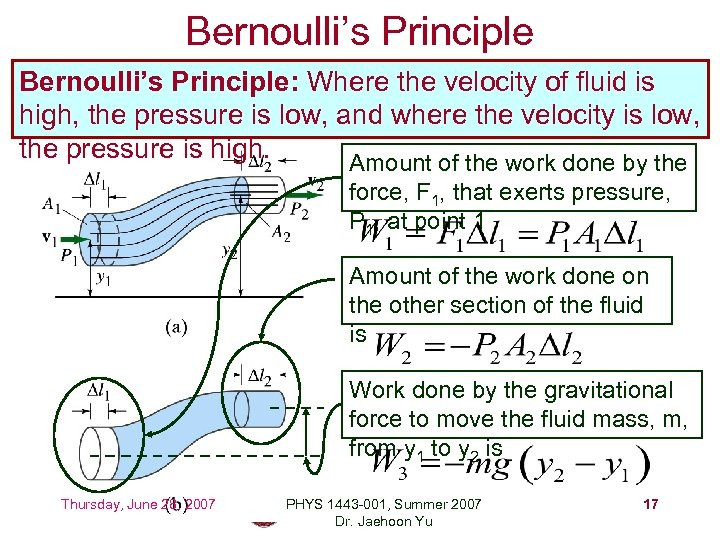Bernoulli’s Principle: Where the velocity of fluid is high, the pressure is low, and where the velocity is low, the pressure is high. Amount of the work done by the force, F 1, that exerts pressure, P 1, at point 1 Amount of the work done on the other section of the fluid is Work done by the gravitational force to move the fluid mass, m, from y 1 to y 2 is Thursday, June 28, 2007 PHYS 1443 -001, Summer 2007 Dr. Jaehoon Yu 17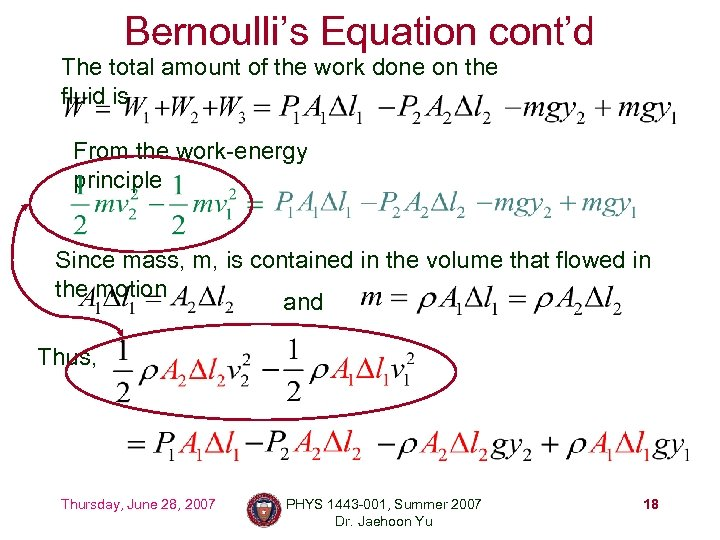Bernoulli’s Equation cont’d The total amount of the work done on the fluid is From the work-energy principle Since mass, m, is contained in the volume that flowed in the motion and Thus, Thursday, June 28, 2007 PHYS 1443 -001, Summer 2007 Dr. Jaehoon Yu 18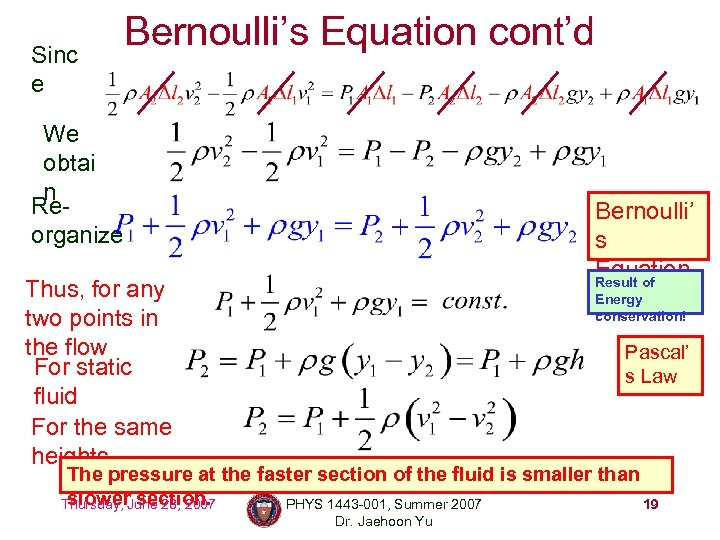Sinc e Bernoulli’s Equation cont’d We obtai n Reorganize Bernoulli’ s Equation Result of Thus, for any two points in the flow For static fluid For the same heights Energy conservation! Pascal’ s Law The pressure at the faster section of the fluid is smaller than slower section. Thursday, June 28, 2007 PHYS 1443 -001, Summer 2007 19 Dr. Jaehoon Yu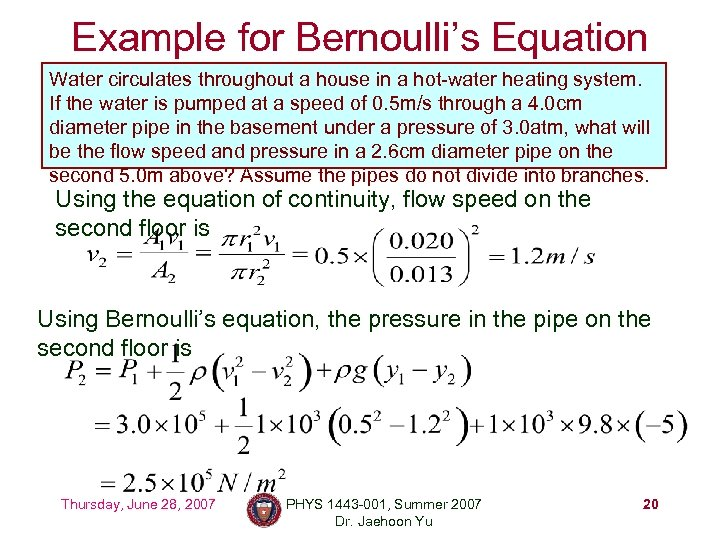Example for Bernoulli’s Equation Water circulates throughout a house in a hot-water heating system. If the water is pumped at a speed of 0. 5 m/s through a 4. 0 cm diameter pipe in the basement under a pressure of 3. 0 atm, what will be the flow speed and pressure in a 2. 6 cm diameter pipe on the second 5. 0 m above? Assume the pipes do not divide into branches. Using the equation of continuity, flow speed on the second floor is Using Bernoulli’s equation, the pressure in the pipe on the second floor is Thursday, June 28, 2007 PHYS 1443 -001, Summer 2007 Dr. Jaehoon Yu 20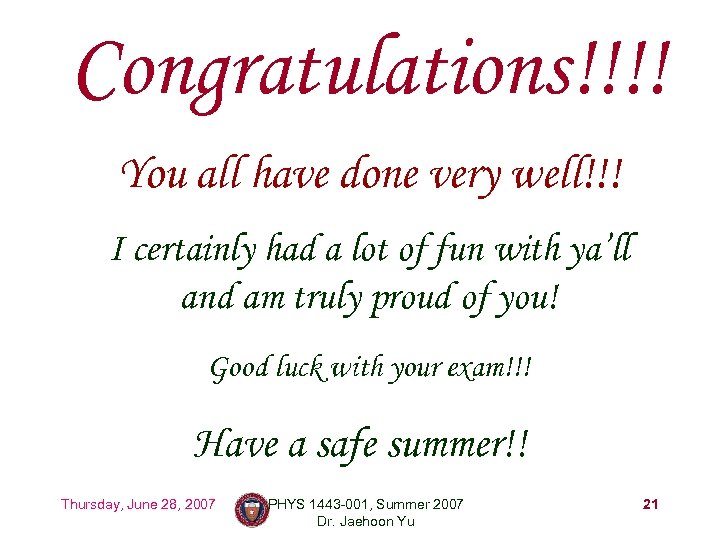Congratulations!!!! You all have done very well!!! I certainly had a lot of fun with ya’ll and am truly proud of you! Good luck with your exam!!! Have a safe summer!! Thursday, June 28, 2007 PHYS 1443 -001, Summer 2007 Dr. Jaehoon Yu 21